# KSEEB Solutions for Class 8 Maths Chapter 7 Rational Numbers Ex 7.3

Students can Download Maths Chapter 7 Rational Numbers Ex 7.3 Questions and Answers, Notes Pdf, KSEEB Solutions for Class 8 Maths helps you to revise the complete Karnataka State Board Syllabus and score more marks in your examinations.

## Karnataka Board Class 8 Maths Chapter 7 Rational Numbers Ex 7.3

1. Name the property indicated in the following:

Question (i)
315+115 = 430
Closure property of addition

Question (ii)
$$\frac{3}{4} \times \frac{9}{5}=\frac{27}{20}$$
Closure property of multiplication.

Question (iii)
5 + 0 = 0 + 5 = 5
0 is the additive identity.

Question (iv)
$$\frac{8}{9} \times 1=\frac{8}{9}$$
1 is the multiplicative identity

Question (v)
$$\frac{8}{17}+\frac{-8}{17}=0$$
$$\frac { -8 }{ 17 }$$

Question (vi)
$$\frac{22}{23} \times \frac{23}{22}=1$$
$$\frac { 23 }{ 22 }$$ is the multiplicative identity

2. Check the commutative property of addition for the following pairs.

Question (i)
$$\frac{102}{201}, \frac{3}{4}$$Question (ii)
$$\frac{-8}{13}, \frac{23}{27}$$Question (iii)
$$\frac{-7}{9}, \frac{-18}{19}$$
a + b = b + a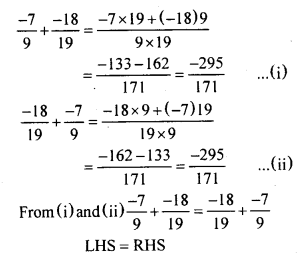3. Check the commutative property of multiplication for the following pairs

Question (i)
$$\frac{22}{45}, \frac{3}{4}$$Question (ii)
$$\frac{-7}{13}, \frac{25}{27}$$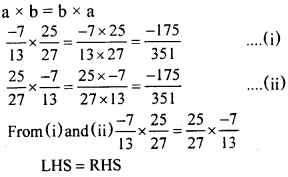Question (iii)
$$\frac{-8}{9}, \frac{-17}{19}$$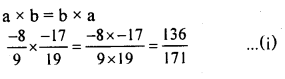4. Check the distributive property for the following triples of rational numbers.

Question (i)
$$\frac{1}{8}, \frac{1}{9}, \frac{1}{10}$$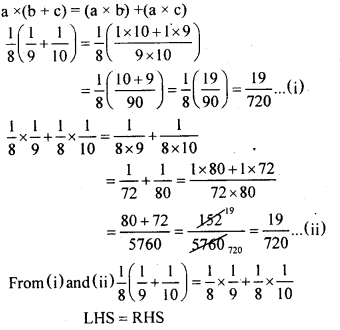Question (ii)
$$\frac{-4}{9}, \frac{6}{5}, \frac{11}{10}$$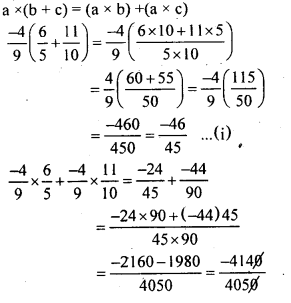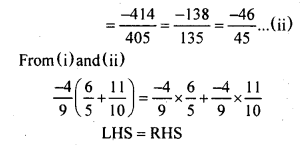Question (iii)
$$\frac{3}{8}, 0, \frac{13}{7}$$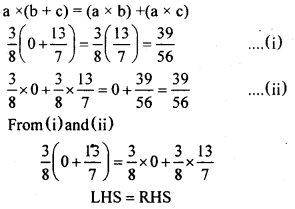5. Find the additive inverse of each of the following numbers.

Question (1)
$$\frac { 8 }{ 5 }$$
$$\frac { -8 }{ 5 }$$

Question (2)
$$\frac { 6 }{ 10 }$$
$$\frac { -6 }{ 10 }$$

Question (3)
$$\frac { -3 }{ 8 }$$
$$\frac { 3 }{ 8 }$$

Question (4)
$$\frac { -16 }{ 3 }$$
$$\frac { 16 }{ 3 }$$

Question (5)
$$\frac { -4 }{ 1 }$$
$$\frac { 4 }{ 1 }$$

6. Find the multiplicative inverse of each of the following numbers.

Question (1)
2
$$\frac { 1 }{ 2 }$$

Question (2)
$$\frac { 6 }{ 11 }$$
$$\frac { 11 }{ 6 }$$

Question (3)
$$\frac { -8 }{ 15 }$$
$$\frac { -15 }{ 8 }$$
$$\frac { 19 }{ 18 }$$
$$\frac { 18 }{ 19 }$$
$$\frac { 1 }{ 1000 }$$
$$\frac { 1000 }{ 1 }$$